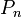# Braid group

## Definition

### In terms of the presentation using Artin braid relations

The braid group on$n$ letters, denoted$B_n$, is defined as follows:$\langle s_1, s_2, \dots, s_{n-1} \mid s_is_{i+1}s_i = s_{i+1}s_is_{i+1} \ \forall \ 1 \le i \le n - 2, s_is_j = s_js_i \ \forall \ |i - j| > 1 \rangle$.

## Facts

There is a natural surjective homomorphism from the braid group$B_n$ to the symmetric group$S_n$, that sends each$s_i$ to the transposition$(i,i+1)$ in$S_n$. One way of seeing this is noting that the presentation of$S_n$ is obtained by tacking on more relations (namely, the relations that each$s_i$ square to the identity) to the relations for$B_n$.

The kernel of this homomorphism is the pure braid group and is denoted$P_n$.$P_n$ is thus a normal subgroup of finite index in$B_n$. The index is$n!$.

## Particular cases

Value of$n$ Value of$n - 1$ (number of generators for the Artin presentation) Braid group$B_n$ Symmetric group$S_n$ Pure braid group$P_n$(kernel of natural homomorphism to symmetric group)
1 0 trivial group trivial group trivial group
2 1 group of integers cyclic group:Z2 group of integers
3 2 braid group:B3 symmetric group:S3 pure braid group:P3
4 3 braid group:B4 symmetric group:S4 pure braid group:P4
5 4 braid group:B5 symmetric group:S5 pure braid group:P5